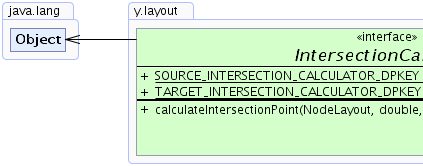Search this API

## y.layout Interface IntersectionCalculator

`public interface IntersectionCalculator`

An `IntersectionCalculator` is responsible for calculating the intersection point between an edge and the visual representation of its source or target node.

This interface is especially useful in conjunction with `PortCalculator` when adjusting the final port locations after the layout is calculated.

`PortCalculator`Field Summary
`static java.lang.Object` `SOURCE_INTERSECTION_CALCULATOR_DPKEY`
A `DataProvider` key for providing an individual intersection calculation for each edge source in the graph
`static java.lang.Object` `TARGET_INTERSECTION_CALCULATOR_DPKEY`
A `DataProvider` key for providing an individual intersection calculation for each edge target in the graph

Method Summary
` YPoint` ```calculateIntersectionPoint(NodeLayout nodeLayout, double xOffset, double yOffset, double dx, double dy)```
Calculates an intersection point between the edge and the given `NodeLayout`.

Field Detail

### SOURCE_INTERSECTION_CALCULATOR_DPKEY

`static final java.lang.Object SOURCE_INTERSECTION_CALCULATOR_DPKEY`
A `DataProvider` key for providing an individual intersection calculation for each edge source in the graph

### TARGET_INTERSECTION_CALCULATOR_DPKEY

`static final java.lang.Object TARGET_INTERSECTION_CALCULATOR_DPKEY`
A `DataProvider` key for providing an individual intersection calculation for each edge target in the graph

Method Detail

### calculateIntersectionPoint

```YPoint calculateIntersectionPoint(NodeLayout nodeLayout,
double xOffset,
double yOffset,
double dx,
double dy)```
Calculates an intersection point between the edge and the given `NodeLayout`.

Implementing classes use a point on the connecting edge segment (specified with an offset to the node's center) along with a direction pointing to the node and calculate the intersection between this line and the node.

Parameters:
`nodeLayout` - the layout information of the node
`xOffset` - the x-coordinate of a point on the last line segment relative to the node's center
`yOffset` - the y-coordinate of a point on the last line segment relative to the node's center
`dx` - the x-component of the normalized direction vector indicating the direction of the segment pointing towards the node
`dy` - the y-component of the normalized direction vector indicating the direction of the segment pointing towards the node
Returns:
the intersection coordinates relative to the node's center or `null` if no such intersection was found